Function Repository Resource:

# ReflexiveGraphQ

Test whether a graph is reflexive

Contributed by: Wolfram Staff (original content by Sriram V. Pemmaraju and Steven S. Skiena)
 ResourceFunction["ReflexiveGraphQ"][g] yields True if the graph g is reflexive and False otherwise.

## Details and Options

A graph is reflexive if each vertex in the graph has an associated self-loop.

## Examples

### Basic Examples (2)

Tuples form a reflexive graph:

 In:=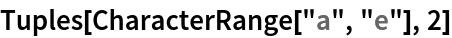Out=In:=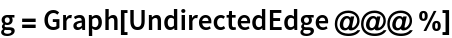Out=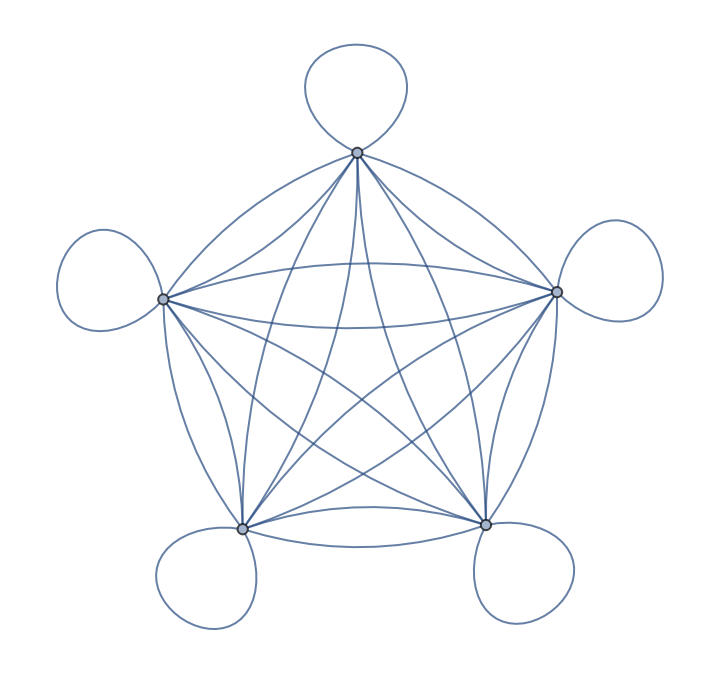In:=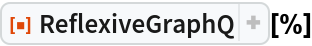Out=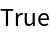After deleting one of its self-loops, the graph is no longer reflexive:

 In:=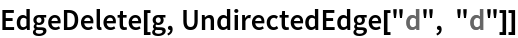Out=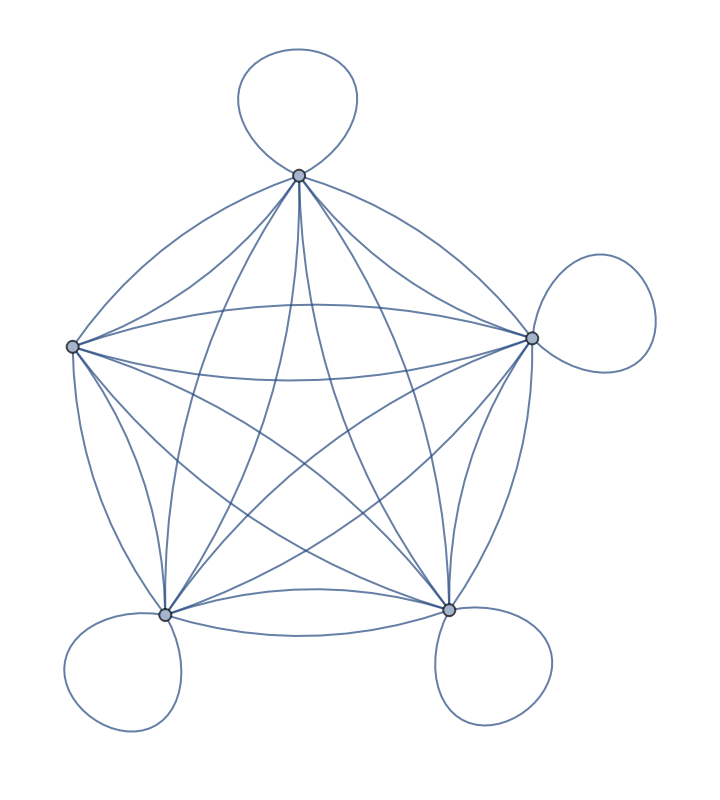In:=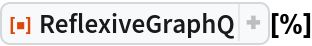Out=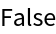The divisibility relation between integers is reflexive since each integer divides itself:

 In:=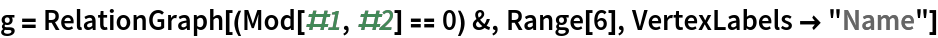Out=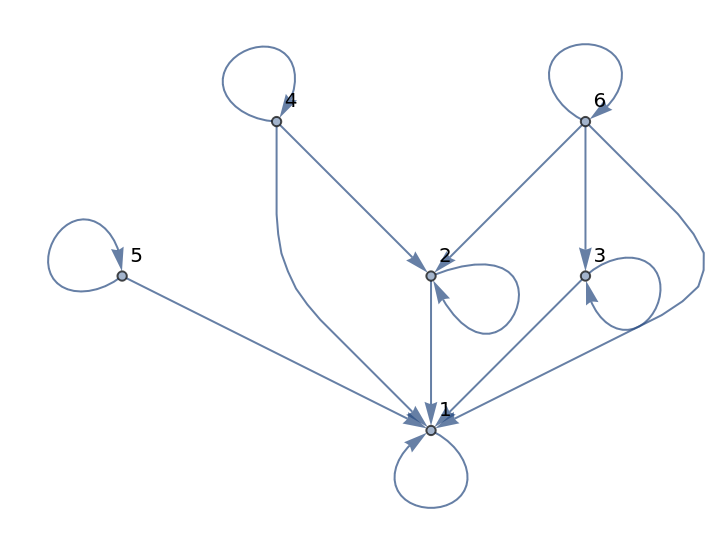In:=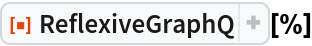Out=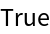## Version History

• 1.0.0 – 01 July 2020